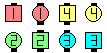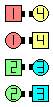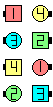Definitions of Square Dance Calls and Concepts

Index -->  Plus  |  A1  |  A2  |  C1  |  C2  |  C3A  |  C3B  |  C4  |  NOL  |
Definitions (Text Only) -->  Plus  |  A1  |  A2  |  C1  |  C2  |  C3A  |  C3B  |  C4  |  NOL  |
 Find call:

Ends \$B\$,GXCf9g\$o\$;\$NJ?9T\$J(B Line, \$B\$^\$?\$OB>\$NE,@Z\$J(B formation \$B\$+\$i(B.

Ends \$B\$O(B, Ends Bend, Turn Thru \$B\$r\$7(B, Centers \$B\$O(B, Face In, Cross Trail Thru \$B\$r\$7\$^\$9(B.

\$BJ?9T\$J(B Line \$B\$+\$i\$O(B, \$BGXCf9g\$o\$;\$N(B Line \$B\$G=*\$o\$j\$^\$9(B. Scramble \$B\$O(B 2 \$B%Q!<%H\$N%3!<%k\$G\$9(B.Scramble\$B\$NA0(B Ends Bend as Centers Face In(1/2)\$B\$N8e(B Ends Turn Thru as Centers Cross Trail Thru\$B\$N8e(B (\$B=*\$o\$j(B)Choreography for ScrambleComments? Questions? Suggestions?

https://www.ceder.net/def/scramble.php?level=C3B&language=japan
01-August-2021 11:04:59